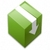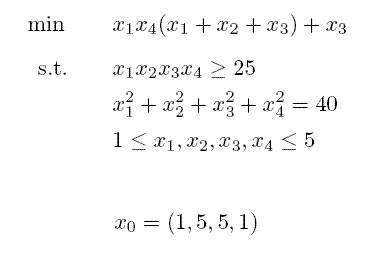## MATLAB Dynamic Optimization ToolboxMATLAB offers a convenient way to access the latest release of APMonitor. The optimization problem is sent to the APMonitor server and results are returned to MATLAB local variables and a web interface. Example applications of nonlinear models with differential and algebraic equations are available for download below or from the following GitHub repository.
 git clone git://github.com/APMonitor/apm_matlabAPM MATLAB with Demo Applications on GitHub

The latest APM MATLAB libraries are attached below. Functionality has been tested with the latest release of MATLAB.APM MATLAB (version 0.7.2) - Released 25 Jan 2017

#### Nonlinear Programming with MATLABHock-Schittkowsky Test Suite #71

#### CSTR: Continuous Stirred Tank ReactorThe APM solution is compared to the ODE15s built-in integrator in MATLAB. Unlike ODE15s, APMonitor allows higher-index DAEs and open-equation format. APMonitor is also a simultaneous equation solver that transforms the differential equations into a Nonlinear Programming (NLP) form. This allows efficient optimization, even for large-scale models.

#### NLC: Nonlinear Control with MATLAB

In another example, the dynamic simulation and control technologies are demonstrated with a simple lag model. The model is composed of one differential equation and one algebraic equation. This model is used as a tutorial to demonstrate the control technology features.This model demonstrates a simulated step change and two controlled step changes. Two different controlled variable error models are compared. At the end of the simulation, a browser window opens to allow the user to view the solution through the web-interface.